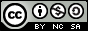- 目录 -
LeetCode 面试题 08.11. 硬币(dp)

 输入: n = 5
输出：2
解释: 有两种方式可以凑成总金额:
5=5
5=1+1+1+1+1


 输入: n = 10
输出：4
解释: 有四种方式可以凑成总金额:
10=10
10=5+5
10=5+1+1+1+1+1
10=1+1+1+1+1+1+1+1+1+1


• 0 <= n (总金额) <= 1000000

## 代码

  1 2 3 4 5 6 7 8 9 10 11 12 13 14 15  class Solution { public: int mod = 1e9 + 7; int v = {1, 5, 10, 25}; int waysToChange(int n) { vector dp(n + 1, 0); dp = 1; for (int i = 0; i < 4; i++) for (int j = v[i]; j <= n; j++) dp[j] = (dp[j] + dp[j - v[i]]) % mod; return dp[n]; } };